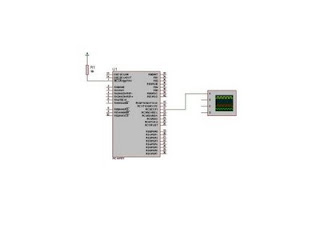Sunday, February 22, 2009

How to use ROM for storing DATA in MikroC Avoid Error Not Enough RAM and Strings problem (const truncated)

Most of the Microcontrolleres having limited RAM, For Avoiding the Errors Not Enough RAM and Strings problem (const truncated) .
You have to move the strings to ROM (FLASH program) memory, and there by save RAM.

In MikroC
if the string is declared as constant - compiler will move it to ROM
This is the way in which const truncated problem can be solved if
great number of strings was used that was located in RAM.

How we can impliment it in MikroC, see the example

First you declare the following

char text; // this is stored in RAM
const char *mesg1="shibuvarkala1234"; // this is stored in ROM
const char *mesg2="CE Attingal"; // this is stored in ROM

here is the function for copying string before the usage ,

// --- Copying strings from ROM to RAM
void strConstCpy(char *dest, const char *source) {
while(*source)
*dest++ = *source++ ;

*dest = 0 ;
}

you have to use the above function to access the strings stored in ROM,

strConstCpy(text,mesg1);
LCD_Out(1,1,text); // Now output is shibuvarkala1234

strConstCpy(text,mesg2);
LCD_Out(1,1,text); // Now output is CE Attingal

Tuesday, February 10, 2009

Pulse Width Modulation or PWM Tutorial using PIC 16F877, Proteus and Mikroc PART-2

Now lets see how to write a code for controlling PWM Module of a PIC Micro Controller using the Mikroc compiler.
MikroC provides a very simple PWM library with 4 functions shown below
• Pwm_Init
• Pwm_Change_Duty
• Pwm_Start
• Pwm_Stop
The pwm_init used for initializing PWM which takes a arguement of frequency in hertz

To Initialize a PWM module at 2KHz do this :Pwm_Init(2000);

Pwm_Start and Pwm_Stop are used for starting and stopping PWM .

Pwm_Change_Duty Changes PWM duty ratio. Parameter duty takes values from 0 to 255. It can be calculate using equation (Percent*255)/100. See 0 is 0%, 127 is 50%, and 255 is 100% duty ratio.

Take a look at the following simple program

// microcontroller : P16F877A
// PWM module is set on RC2 Pin No 17.

unsigned short i;

void main() {
PORTC = 00; // Set PORTC to \$FF
TRISC = 0; // PORTC is output
Pwm_Init(5000); // Initialize PWM module

Pwm_Start(); // Start PWM

while (1) { // Endless loop
for(i=0;i<=255;i++)

{ Pwm1_Change_Duty(i);

delay_ms(10); }

} }This program creates a pwm on pin17 of PIC16F877A .Hope you found this tutorial useful .If you have any doubts about the program .Leave a comment . click to enlarge figure. set CRO channel A Volts to 1 V and time/div to 50us

Pulse Width Modulation or PWM Tutorial using PIC 16F877, Proteus and Mikroc PART-1Pulse width Modulation or PWM is one of the powerful techniques used in todays control systems.
It is used for speed control of motors, used for measurement , communication and power control.

Pulse-width Modulation is achieved with the help of a squarewave whose duty cycle (ON time vs OFF time) is changed to get a varying voltage output as a result of average value of waveform. See Picture

Consider a square wave shown in the figure above.

TON is the time for which the output is high and ToFF is time for which output is low. Let Ttotal be time period of the wave such that, Ttotal = TON +ToFF

Duty cycle of a squarewave is defined as
D = TON / (TON+ToFF)= TON/Ttotal

See output voltage varies with duty cycle

VOUT = D x VIN

VOUT = (TON/Ttotal) x VIN

we see from the final equation the output voltage can be directly varied by varying the TON value.

If TON is Zero , VOUT is also Zero.

Friday, February 6, 2009

How to Read and Write Data into EEPROM using MikroC

This is an Example program for Demonstrating read and write Data into EEPROM of a PIC Microcontroller (Pic16F877A have 256 Bytes EEPROM),This program first writes 0xAA into the address 0x00 and the reads and displays it in PORTB . You don't need external EEPROM for storing small user datas.

Mikroc Having a EEPROM library .There are only two functions in this library
• Eeprom_Write

First function Eeprom_Read returns an integer value from the specified address. The following is the syntax .

Second Function Eeprom_Write takes two parameters address and data .

See syntax given below .

void Eeprom_Write(unsigned int address, unsigned short data);

See the Example program

unsigned short data = 0xAA, address=0x00;

void main() {
PORTB = 0x00;
TRISB = 0x00;

//writes 0xAA into the location 0x00

//insert 20 or 30 ms delay between every read and write cycle
Delay_ms(20);

// the following delay is just for making a feel
Delay_ms(1000);

//reads the data from 0x00 and send it to PORTB

Delay_ms(1000);
}

Thursday, February 5, 2009

Digital Clock using PIC16F877A and DS1307 RTC Code in MikroC

This picture shows the Circuit Simulated in ProteusMikroC Code for RTC DS1307 and PIC16F877A in Mikroc here
http://rapidshare.com/files/194319667/ds1307_Mikroc_shibu.c.html